# Maximum sum of non-adjacent elements (DP 5)

In this article we will solve the problem: Maximum sum of non-adjacent elements (DP 5)

Problem Statement:

Given an array of ‘N’  positive integers, we need to return the maximum sum of the subsequence such that no two elements of the subsequence are adjacent elements in the array.

Note: A subsequence of an array is a list with elements of the array where some elements are deleted ( or not deleted at all) and the elements should be in the same order in the subsequence as in the array.

Examples:

`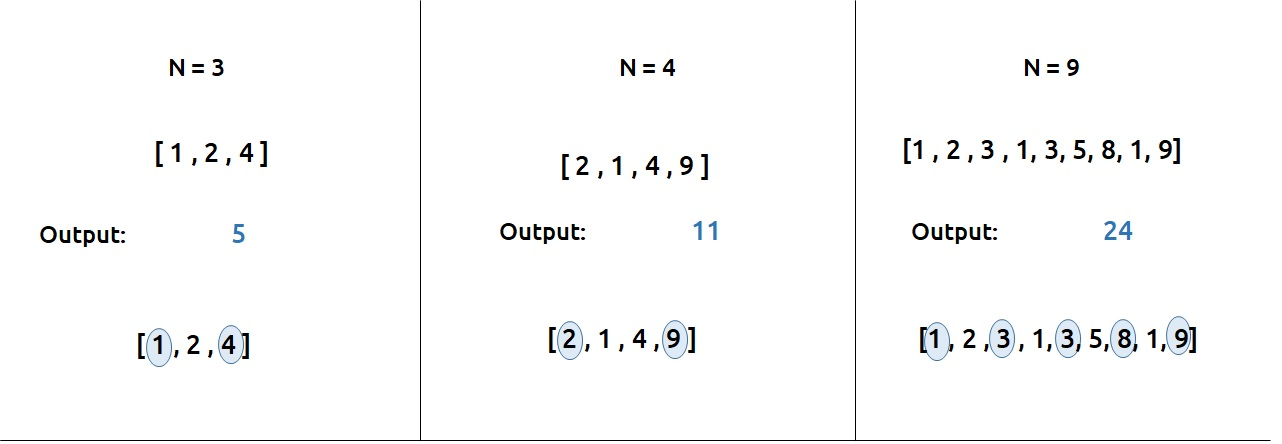`

Pre-req: Recursion, Dynamic Programming Introduction

### Solution :

As we need to find the sum of subsequences, one approach that comes to our mind is to generate all subsequences and pick the one with the maximum sum.

To generate all the subsequences, we can use the pick/non-pick technique. This technique can be briefly explained as follows:

• At every index of the array, we have two options.
• First, to pick the array element at that index and consider it in our subsequence.
• Second, to leave the array element at that index and not to consider it in our subsequence.

A more detailed explanation of this technique is taught in Recursion on subsequences. Readers are highly advised to watch that video.

First, we will try to form the recursive solution to the problem with the pick/non-pick technique. There is one more catch, the problem wants us to have only non-adjacent elements of the array in the subsequence, therefore we need to address that too.

Steps to form the recursive solution

We will use the steps mentioned in the article Dynamic Programming Introduction in order to form our recursive solution.

Step 1: Form the function in terms of indexes:

• We are given an array which can be easily thought of in terms of indexes.
• We can define our function f(ind) as : Maximum sum of the subsequence starting from index 0 to index ind.
• We need to return f(n-1) as our final answer.

Step 2: Try all the choices to reach the goal.

As mentioned earlier we will use the pick/non-pick technique to generate all subsequences. We also need to take care of the non-adjacent elements in this step.

• If we pick an element then, pick = arr[ind] + f(ind-2). The reason we are doing f(ind-2) is because we have picked the current index element so we need to pick a non-adjacent element so we choose the index ‘ind-2’ instead of ‘ind-1’.
• Next we need to ignore the current element in our subsequence. So nonPick= 0 + f(ind-1). As we don’t pick the current element, we can consider the adjacent element in the subsequence.

Our pseudocode till this step will be: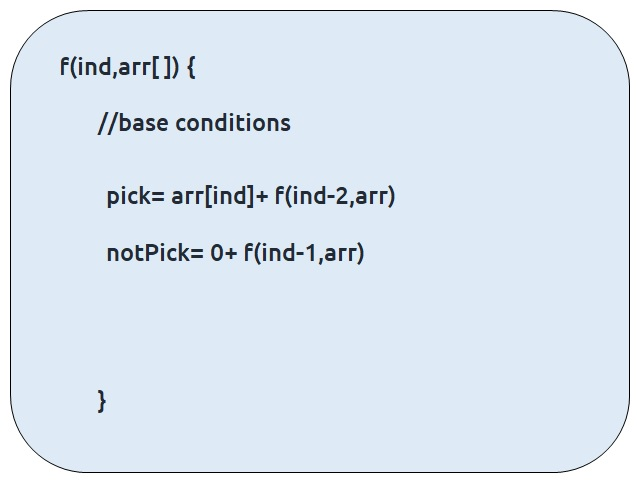Step 3: Take the maximum of all the choices

As the problem statement asks to find the maximum subsequence total, we will return the maximum of two choices of step2.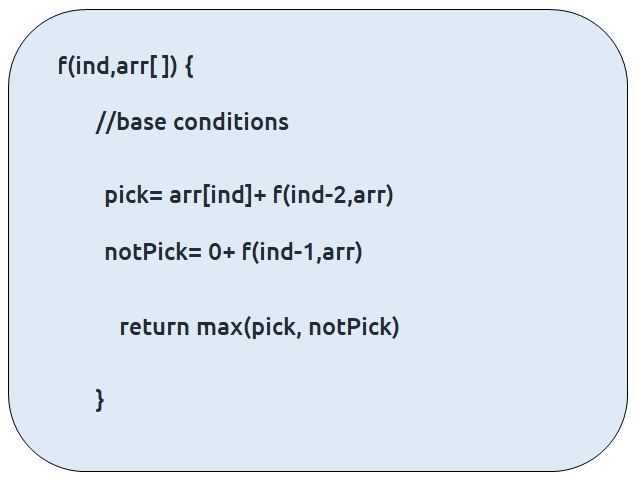Base Conditions

The base conditions for the recursive function will be as follows:

• If ind=0, then we know to reach at index=0, we would have ignored the element at index = 1. Therefore, we can simply return the value of arr[ind] and consider it in the subsequence.
• If ind<0, this case can hit when we call f(ind-2) at ind=1. In this case we want to return to the calling function so we simply return 0 so that nothing is added to the subsequence sum.

Our final pseudo-code will be: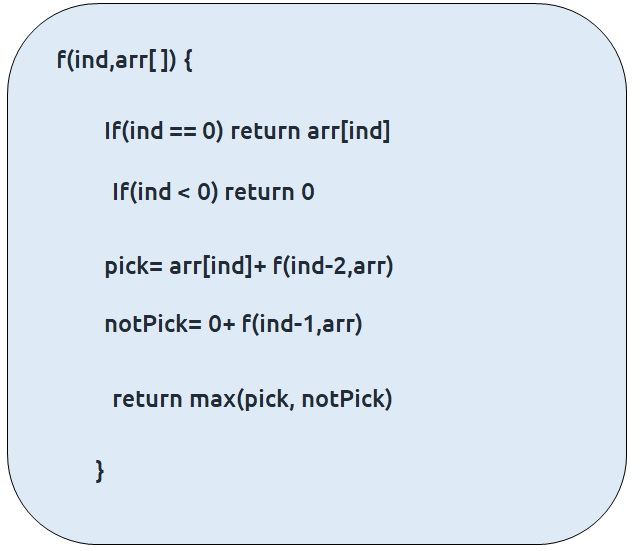Once we form the recursive solution, we can use the approach told in Dynamic Programming Introduction to convert it into a dynamic programming one.

Memoization approach

If we observe the recursion tree, we will observe a number of overlapping subproblems. Therefore the recursive solution can be memoized to reduce the time complexity.

Recursion tree diagram: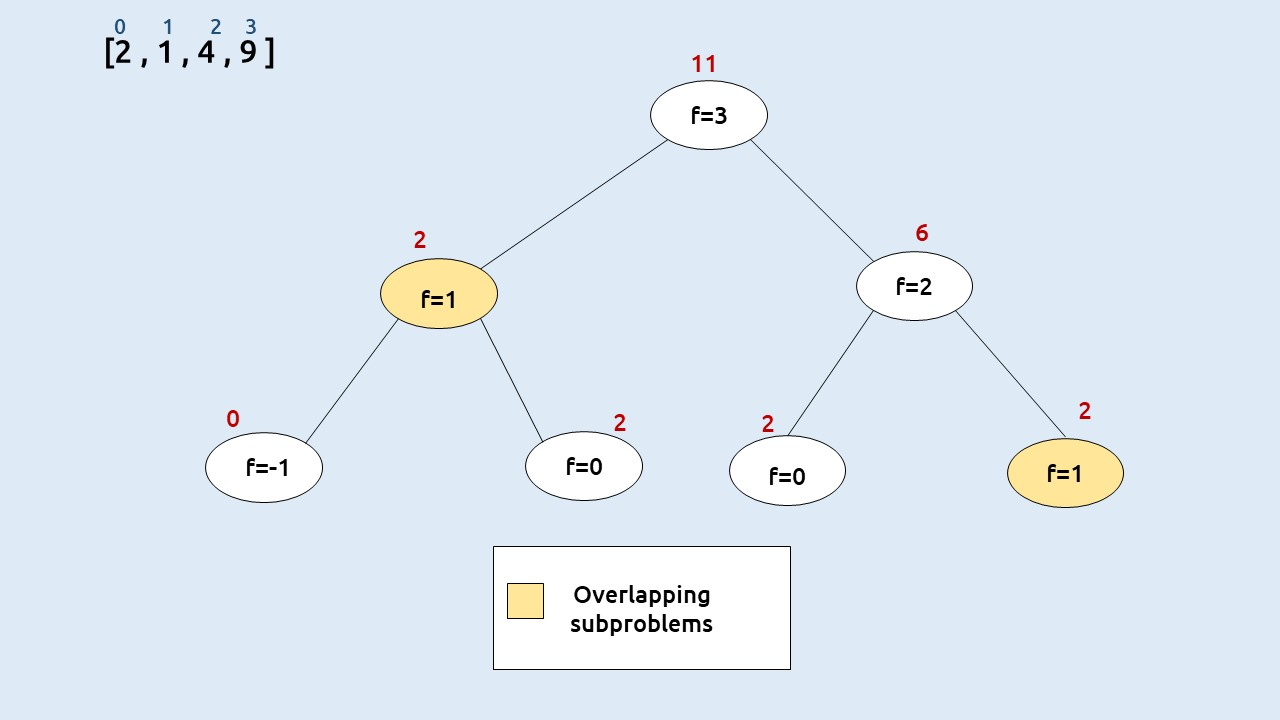Note: To watch a detailed dry run of this approach, please watch the video attached below

Steps to convert Recursive code to memoization solution:

• Create a dp[n] array initialized to -1.
• Whenever we want to find the answer of a particular value (say n), we first check whether the answer is already calculated using the dp array(i.e dp[n] != -1 ). If yes, simply return the value from the dp array.
• If not, then we are finding the answer for the given value for the first time, we will use the recursive relation as usual but before returning from the function, we will set dp[n] to the solution we get.

Code:

## C++ Code

``````#include <bits/stdc++.h>

using namespace std;

int solveUtil(int ind, vector<int>& arr, vector<int>& dp){

if(dp[ind]!=-1) return dp[ind];

if(ind==0) return arr[ind];
if(ind<0)  return 0;

int pick= arr[ind]+ solveUtil(ind-2, arr,dp);
int nonPick = 0 + solveUtil(ind-1, arr, dp);

return dp[ind]=max(pick, nonPick);
}

int solve(int n, vector<int>& arr){
vector<int> dp(n,-1);
return solveUtil(n-1, arr, dp);
}

int main() {

vector<int> arr{2,1,4,9};
int n=arr.size();
cout<<solve(n,arr);

}
``````

Output: 11

Time Complexity: O(N)

Reason: The overlapping subproblems will return the answer in constant time O(1). Therefore the total number of new subproblems we solve is ‘n’. Hence total time complexity is O(N).

Space Complexity: O(N)

Reason: We are using a recursion stack space(O(N)) and an array (again O(N)). Therefore total space complexity will be O(N) + O(N) ≈ O(N)

## Java Code

``````import java.util.*;
public class Main{
static int solveUtil(int ind, int[] arr, int[] dp){
if(ind<0)  return 0;
if(ind==0) return arr[ind];
if(dp[ind]!=-1) return dp[ind];
int pick= arr[ind]+ solveUtil(ind-2, arr,dp);
int nonPick = 0 + solveUtil(ind-1, arr, dp);

return dp[ind]=Math.max(pick, nonPick);
}

static int solve(int n,int[] arr){
int dp[]=new int[n];
Arrays.fill(dp,-1);
return solveUtil(n-1, arr, dp);
}

public static void main(String args[]) {

int arr[]={2,1,4,9};
int n=arr.length;
System.out.println(solve(n,arr));
}
}
``````

Output: 11

Time Complexity: O(N)

Reason: The overlapping subproblems will return the answer in constant time O(1). Therefore the total number of new subproblems we solve is ‘n’. Hence total time complexity is O(N).

Space Complexity: O(N)

Reason: We are using a recursion stack space(O(N)) and an array (again O(N)). Therefore total space complexity will be O(N) + O(N) ≈ O(N)

Tabulation approach

• Declare a dp[] array of size n.
• First initialize the base condition values, i.e dp as 0.
• Set an iterative loop which traverses the array( from index 1 to n-1) and for every index calculate pick  and nonPick
•  And then we can set dp[i] = max (pick, nonPick)

Code:

## C++ Code

``````#include <bits/stdc++.h>

using namespace std;

int solveUtil(int n, vector<int>& arr, vector<int>& dp){

dp= arr;

for(int i=1 ;i<n; i++){
int pick = arr[i];
if(i>1)
pick += dp[i-2];
int nonPick = 0+ dp[i-1];

dp[i]= max(pick, nonPick);
}

return dp[n-1];
}

int solve(int n, vector<int>& arr){
vector<int> dp(n,-1);
return solveUtil(n, arr, dp);
}

int main() {

vector<int> arr{2,1,4,9};
int n=arr.size();
cout<<solve(n,arr);

}
``````

Output: 11

Time Complexity: O(N)

Reason: We are running a simple iterative loop

Space Complexity: O(N)

Reason: We are using an external array of size ‘n+1’.

## Java Code

``````import java.util.*;
class TUF{
static int solveUtil(int n, int[] arr, int[] dp){

dp= arr;

for(int i=1 ;i<n; i++){
int pick = arr[i];
if(i>1)
pick += dp[i-2];
int nonPick = 0+ dp[i-1];

dp[i]= Math.max(pick, nonPick);
}

return dp[n-1];
}

static int solve(int n, int[] arr){
int dp[]=new int[n];
Arrays.fill(dp,-1);
return solveUtil(n, arr, dp);
}

public static void main(String args[]) {

int arr[]={2,1,4,9};
int n=arr.length;
System.out.println(solve(n,arr));
}
}
``````

Output: 11

Time Complexity: O(N)

Reason: We are running a simple iterative loop

Space Complexity: O(N)

Reason: We are using an external array of size ‘n+1’.

Part 3: Space Optimization

If we closely look the values required at every iteration,

dp[i] , dp[i-1] and  dp[i-2]

we see that for any i, we do need only the last two values in the array. So is there a need to maintain a whole array for it?

The answer is ‘No’. Let us call dp[i-1] as prev and dp[i-2] as prev2. Now understand the following illustration.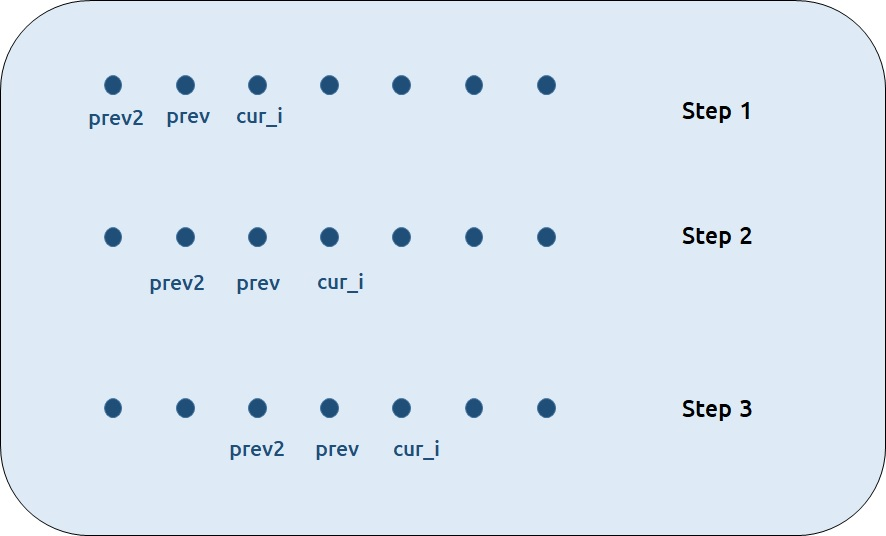• Each iteration’s cur_i and prev becomes the next iteration’s prev and prev2 respectively.
• Therefore after calculating cur_i, if we update prev and prev2 according to the next step, we will always get the answer.
• After the iterative loop has ended we can simply return prev as our answer.

Code:

## C++ Code

``````#include <bits/stdc++.h>

using namespace std;

int solve(int n, vector<int>& arr){
int prev = arr;
int prev2 =0;

for(int i=1; i<n; i++){
int pick = arr[i];
if(i>1)
pick += prev2;
int nonPick = 0 + prev;

int cur_i = max(pick, nonPick);
prev2 = prev;
prev= cur_i;

}
return prev;
}

int main() {

vector<int> arr{2,1,4,9};
int n=arr.size();
cout<<solve(n,arr);

}
``````

Output: 11

Time Complexity: O(N)

Reason: We are running a simple iterative loop

Space Complexity: O(1)

Reason: We are not using any extra space.

## Java Code

``````import java.util.*;
class TUF{
static int solve(int n, int[] arr){

int prev = arr;
int prev2 =0;

for(int i=1; i<n; i++){
int pick = arr[i];
if(i>1)
pick += prev2;
int nonPick = 0 + prev;

int cur_i = Math.max(pick, nonPick);
prev2 = prev;
prev= cur_i;

}
return prev;
}
public static void main(String args[]) {

int arr[]={2,1,4,9};
int n=arr.length;
System.out.println(solve(n,arr));
}
}
``````

Output: 11

Time Complexity: O(N)

Reason: We are running a simple iterative loop

Space Complexity: O(1)

Reason: We are not using any extra space.

Special thanks to Anshuman Sharma for contributing to this article on takeUforward. If you also wish to share your knowledge with the takeUforward fam, please check out this article